Clutch Prep is now a part of Pearson
Ch.3 - Chemical ReactionsWorksheetSee all chapters

# Theoretical Yield (IGNORE)

See all sections
Sections
Empirical Formula
Molecular Formula
Combustion Analysis
Combustion Apparatus
Polyatomic Ions
Naming Ionic Compounds
Writing Ionic Compounds
Naming Ionic Hydrates
Naming Acids
Naming Molecular Compounds
Balancing Chemical Equations
Stoichiometry
Limiting Reagent
Percent Yield
Mass Percent
Functional Groups in ChemistryJules Bruno

In a chemical reaction that involves reacting molecules the maximum amount of product formed from the limiting reactant is called the theoretical yield.

In order to calculate the theoretical yield of a chemical reaction we must deal with stoichiometry and have a balanced equation.

First, always make sure to check to see if your chemical equation is balanced.Unbalanced Chemical Equation

Once you’ve balanced your chemical equation you can start calculations dealing with stoichiometry.Balanced Chemical Equation

When dealing with stoichiometry you can utilize the stoichiometric chart.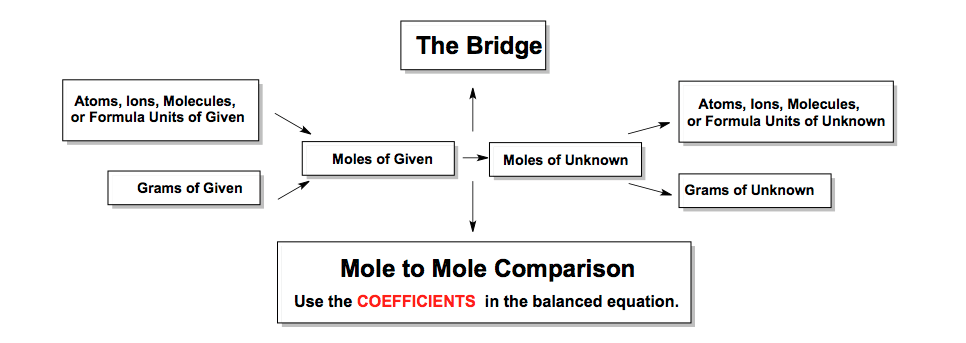The Stoichiometric Chart

When you are given a balanced equation and information on more than one reactant or starting material you will utilize the stoichiometric chart in order to calculate your theoretical yield.

Let’s Get Started!

The amount of reagents or reactants will be used to determine the maximum amount of product possible.

For example, "If 25.0 g of hydrogen gas reacts with 35.0 g of nitrogen gas to produce ammonia, what is the amount of product (in grams) formed?Balanced Chemical EquationSTEP 1: Calculate the molecular weights of H­2, N2 and NH3 with the atomic masses of the elements   taken from the periodic table.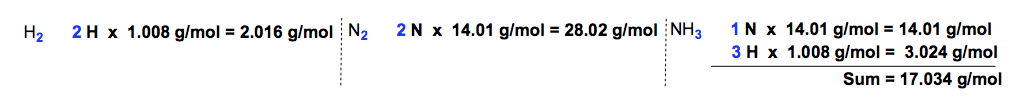Calculating Molar MassesSTEP 2: Convert the grams of reactants into moles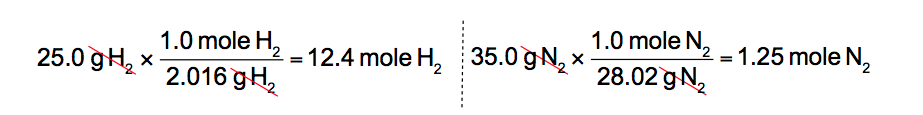Grams to moles conversions

STEP 3: Convert the moles of reactants by doing mole-to-mole comparisons.Balanced-Chemical-EquationDuring this step we use the coefficients from the balanced equation.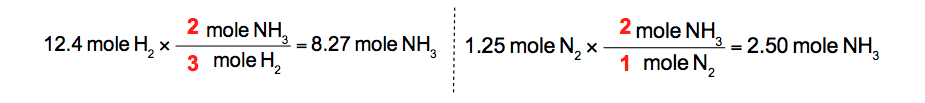Mole to mole ratio

STEP 4: Convert the moles of product into grams of product.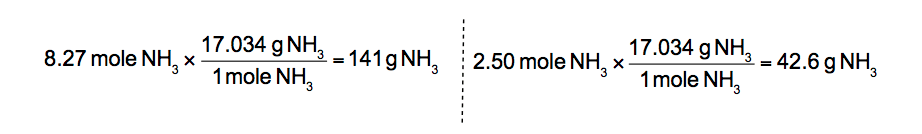Moles to grams conversions

STEP 5: Determine the theoretical yield.

By comparing the amounts of product we state that the theoretical yield, sometimes referred to as the 100% yield, represents the smaller amount. Therefore the theoretical yield is 42.6 g NH3

What’s next?

After determining the theoretical yield of a chemical reaction we can branch out to other stoichiometric operations.

We can calculate percent yield by using theoretical yield and actual yield. In addition we can deal with solution chemistry by relating molarity to mL, L, moles and stoichiometry.Jules Bruno

Jules felt a void in his life after his English degree from Duke, so he started tutoring in 2007 and got a B.S. in Chemistry from FIU. He’s exceptionally skilled at making concepts dead simple and helping students in covalent bonds of knowledge.